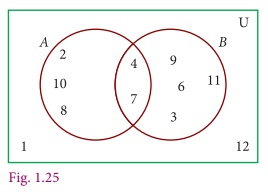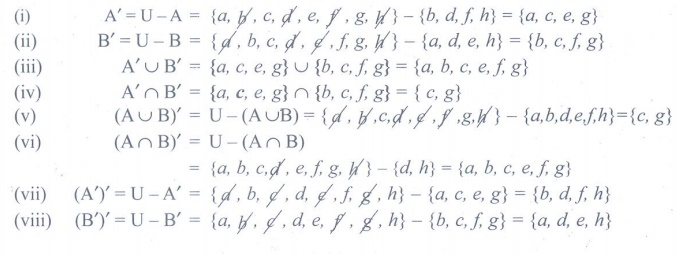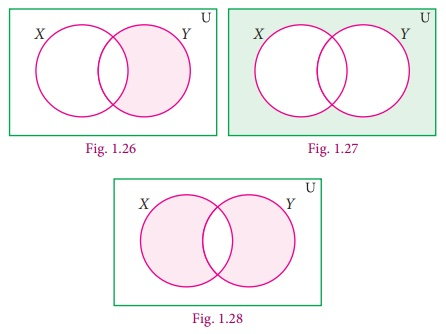Home | | Maths 9th std | Exercise 1.3: Set Operations

# Exercise 1.3: Set Operations

Maths : Set Language : Book Back, Exercise, Example Numerical Question with Answers, Solution : Exercise 1.3: Set Operations

Exercise 1.3

1. Using the given Venn diagram, write the elements of(i) A (ii) B (iii) AŌł¬B (iv) AŌł®B (v) AŌĆōB (vi) BŌĆōA (vii) AŌĆ▓ (viii) BŌĆ▓ (ix) U2. Find AŌł¬B, AŌł®B, AŌĆōB and BŌĆōA for the following sets.

(i) A = {2, 6, 10, 14} and B={2, 5, 14, 16}

(ii) A = {a, b, c, e, u} and B={a, e, i, o, u}

(iii) A = {x : x Ōłł N, x Ōēż 10} and B={x : x ŌłłW, x < 6}

(iv) A = Set of all letters in the word ŌĆ£mathematicsŌĆØ and

B = Set of all letters in the word ŌĆ£geometryŌĆØ3. If U={a, b, c, d, e, f, g, h}, A={b, d, f, h} and B={a, d, e, h}, find the following sets.

(i) AŌĆ▓ (ii) BŌĆ▓ (iii) AŌĆ▓Ōł¬BŌĆ▓ (iv) AŌĆ▓Ōł®BŌĆ▓ (v) (AŌł¬B)ŌĆ▓ (vi) (AŌł®B)ŌĆ▓ (vii) (AŌĆ▓)ŌĆ▓ (viii) (BŌĆ▓)ŌĆ▓4. Let U={0, 1, 2, 3, 4, 5, 6, 7}, A={1, 3, 5, 7} and B={0, 2, 3, 5, 7}, find the following sets.

(i) AŌĆ▓ (ii) BŌĆ▓ (iii) AŌĆ▓Ōł¬BŌĆ▓ (iv)AŌĆ▓Ōł®BŌĆ▓ ( v)(AŌł¬B)ŌĆ▓ (vi) (AŌł®B)ŌĆ▓ (vii) (AŌĆ▓)ŌĆ▓ (viii) (BŌĆ▓)ŌĆ▓5. Find the symmetric difference between the following sets.

(i) P = {2, 3, 5, 7, 11} and Q={1, 3, 5, 11}

(ii) R = {l, m, n, o, p} and S = {j, l, n, q}

(iii) X = {5, 6, 7} and Y={5, 7, 9, 10}6. Using the set symbols, write down the expressions for the shaded region in the following7. Let A and B be two overlapping sets and the universal set be U. Draw appropriate Venn diagram for each of the following,

(i) AŌł¬B (ii) AŌł®B (iii) (AŌł®B)ŌĆ▓ (iv) (BŌĆōA)ŌĆ▓ (v) AŌĆ▓Ōł¬BŌĆ▓ (vi) AŌĆ▓Ōł®BŌĆ▓ (vii) What do you observe from the Venn diagram (iii) and (v)?Tags : Numerical Problems with Answers, Solution | Set Language | Maths , 9th Maths : UNIT 1 : Set Language
Study Material, Lecturing Notes, Assignment, Reference, Wiki description explanation, brief detail
9th Maths : UNIT 1 : Set Language : Exercise 1.3: Set Operations | Numerical Problems with Answers, Solution | Set Language | Maths